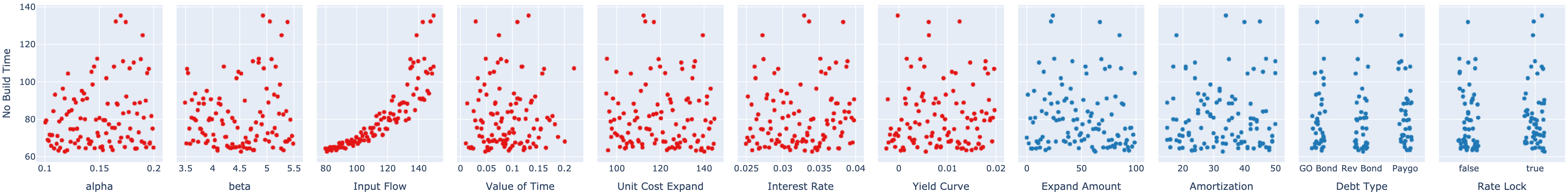:

import emat
emat.versions()

emat 0.5.2, plotly 4.14.3


# Scatter Plot Matrix¶

Once a series of experiments has been conducted for a core model, it is suggested that an analyst review the results to confirm that the model is behaving as expected. TMIP-EMAT provides some visualization tools to review results in a graphical manner, which is a generally convenient method to review this experimental data.

To demonstrate these tools, we will use the Road Test example model included in TMIP-EMAT. We can quickly construct and run a design of experiments to exercise this model and populate some results to visualize:

:

import emat.examples
design = model.design_experiments()
results = model.run_experiments(design)


Given this set of experimental results, we can display a scatter plot matrix to see the results. This is a collection of two-dimensional plots, each showing a contrast between two factors, typically an input parameter (i.e.  an uncertainty or a policy lever) and an output performance measure, although it is also possible to plot inputs against inputs or outputs against outputs.

The display_experiments function in the emat.analysis sub-package can automatically create a scatter plot matrix that crosses every parameter with every measure, simply by providing the scope and the results. By default, plots that display levers are shown in blue, plots that show uncertainties are in red.

:

from emat.analysis import display_experiments
display_experiments(scope, results)


#### No Build Time